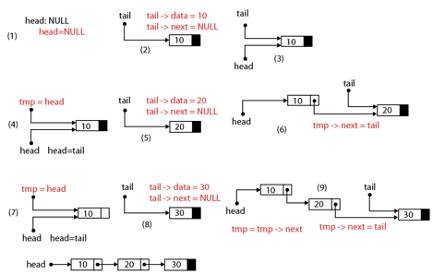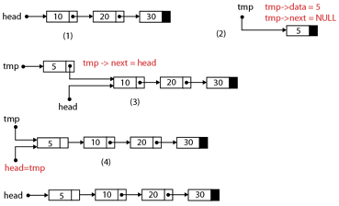Is a data structure that makes it easy to rearrange data without having to move data in memory.

A linear collection of specially designed data elements, called nodes, linked to one another by means of pointers.

## Parts of node

1. Contains the information of the element (data).
2. Contains the address of the next node in the linked list (next).

1. Linked list are dynamic data structure. That is, they can grow or shrink during the execution of a program.
2. Efficient memory utilization: In linked list (or dynamic)representation, memory is not pre-allocated. Memory is allocated whenever it is required. And it is deallocated (or removed) when it is not needed.
3. Insertion and deletion are easier and efficient. Linked list provides flexibility in inserting a data item at a specified position and deletion of a data item from the given position.
4. Many complex applications can be easily carried out with linked list.

1. More memory: to store an integer number, a node with integer data and address field is allocated. That is more memory space is needed.
2. Access to an arbitrary data item is little bit cumbersome and also time consuming.

## Linked List Operations

Creation

Create a linked list. Once a linked list is created with one node, insertion operation can be used to add more elements in a node.

Insertion

Insert a new node at any specified location in the linked list (beginning, end, or specified position between linked list).

Deletion

Delete an item (or node) from the linked list (beginning, end, specified location of the linked list)

Traversing

Process of going through all the nodes from one end to another end of the linked list. In singly linked list we can visit from left to right, forward traversing is nodes only. In doubly linked list forward and backward traversing is possible.

Searching

Search values from the linked list.

Concatenation

Appending the second list to the end of the first list.

## Types of linked list

Enables a program to move through the list in one direction, which is usually from the front of the list moving to the end of the list.Creation (3 nodes with data value of 10, 20, and 30)Insertion (insert node with data value of 5 at the beginning)

## Linked list sample program

```
#include <iostream>
using namespace std;

struct node{
int data;
node *next;
};

node *start;

void createList(int d);
void display();

int main()
{
start = NULL;
int n,m;

cout << "\n\nHow many nodes you want: ";
cin >> n;
for(int i=0;i<n;i++) {
cout << "\nEnter the element: ";
cin >> m;
createList(m);
}

display();

display();
cin.ignore().get();
return 0;
}

{
node *tmp;
tmp = new node;
tmp -> data = d;
tmp -> next = start;
start = tmp;
}

void display()
{
node *q;
if(start==NULL){
cout << "\n\nList is empty";
return;
}
q=start;
cout << "\n\nList is: ";
while(q!=NULL){
cout << q->data << " ";
q=q->next;
}
cout << "\n";
}

void createList(int d)
{
node *q, *tmp;
tmp = new node;
tmp -> data = d;
tmp -> next = NULL;
if(start==NULL) {
start = tmp;
} else {
q = start;
while(q->next!=NULL)
q = q->next;
q->next = tmp;
}
}
```

## Singly Linked List

```
/*
Program: Singly Linked Queue

*/

#include <iostream>
#include <iomanip>
using namespace std;

struct node{
int data;
node *next;

void createlist(int);
void display();
void del(int);
void delfirstelem();
void count();
void reverse();
void search(int);

int main()
{
while(1)
{
int choice;
system("cls");
cout << " - Create a list\n";
cout << " - Add at the beginning\n";
cout << " - Add after\n";
cout << " - Delete\n";
cout << " - Display\n";
cout << " - Count\n";
cout << " - Reverse\n";
cout << " - Search\n";
cout << " - Exit\n";
cout << "===================\n";
cout << "Enter your choice: ";
cin >> choice;
int n, num, pos;
switch(choice){
case 1:
cout << "\nHow many node you want: ";
cin >> n;
cout << "\nEnter " << n << " element(s)\n";
for(int i=0;i<n;i++)
{
cin >> num;
createlist(num);
}
display();
break;
case 2:
cout << "\nEnter a number (add at beginning)";
cin >> num;
display();
break;
case 3:
cout << "\nEnter the value and position of your element: ";
cin >> num >> pos;
display();
break;
case 4:
cout << "\nEnter the value to be deleted from the list: ";
cin >> num;
del(num);
//cout << "delete first element ";
//delfirstelem();
system("pause>0");
display();
break;
case 5:
display();
break;
case 6:
count();
break;
case 7:
reverse();
display();
break;
case 8:
cout << "\nEnter the value to search: ";
cin >> num;
search(num);
break;
case 9:
exit(1);
default:
cout << "\nWrong choice!\n";
}
cout << endl;
system("pause");
}
return 0;
}

void delfirstelem()
{
node *tmp;
delete tmp;
}

//creation
void createlist(int n)
{
node *p, *tail;
tail = new node;
tail->data = n;
tail->next = NULL;
else
{
while(p->next!=NULL)
p=p->next;
p->next = tail;
}
}

{
node *tmp;
tmp = new node;
tmp->data = n;
}

void addafter(int n,int pos)
{
node *tmp, *p;

{
cout << "You have to create first the linked list\n";
return;
}

for(int i=0;i<pos-1;i++)
{
p=p->next;
if(p==NULL)
{
cout << "There are less than "<<pos<<" elements";
return;
}
}
tmp = new node;
tmp->next = p ->next;
tmp->data = n;
p->next = tmp;

}

void del(int n)
{
node *tmp,*p;
//delete at the beginning
{
cout << "You have to create first the linked list\n";
return;
}
{
delete tmp;
return;
}
//delete in between
while(p->next->next!=NULL)
{
if(p->next->data == n)
{
tmp = p->next;
p->next = tmp->next;
delete tmp;
return;
}
p=p->next;
}
if(p->next->data == n)
{
tmp = p->next;
delete tmp;
p->next=NULL;
return;
}
cout << "\nElement " << n << " not found\n";
}

//count
void count()
{
node *p;
int cnt=0;
while(p!=NULL)
{
p=p->next;
cnt++;
}
cout << "There are " << cnt <<" elements is the list\n";
}

void reverse()
{
node *p1, *p2, *p3;
//for one element only
return;
p2=p1->next;
p3=p2->next;
p2->next=p1;
p1->next=NULL;

while(p3!=NULL)
{
p1=p2;
p2=p3;
p3=p3->next;
p2->next=p1;
}
}

void search(int n)
{
node *p;
int pos=1;
while(p!=NULL)
{
if(p->data==n)
{
cout << "The element value "<<n<<" found at position "<< pos;
return;
}
pos++;
p=p->next;
}
cout << "The element value "<<n<<" is not found\n";
}

//display
void display()
{
node *p;
{
cout << "List is empty!\n";
return;
}
cout << endl;
cout << setw(20) << "POINTER" << setw(20) << "DATA" << setw(20) << "NEXT" << endl;
while(p!=NULL)
{
cout << setw(20) << p << setw(20) << p->data << setw(20) << p->next << endl;
p = p->next;
}
cout << endl;
}
```

## Doubly Linked list

```#include <iostream>
#include <iomanip>
using namespace std;

struct node{
int data;
node *next;
node *prev;
}*start;

//typedef node *NODE;

void display()
{
node *p;
if(start==NULL)
{
cout << "List is empty!\n";
return;
}
p=start;
cout << endl;
cout << setw(10) << "ADDRESS" << setw(15) << "PREV" <<setw(15) << "DATA" << setw(20) << "NEXT" << endl;
while(p!=NULL)
{
cout << setw(10) << p << setw(15) << p->prev <<setw(15) << p->data << setw(20) << p->next << endl;
p = p->next;
}
cout << endl;
}
void createlist(int n)
{
node *p, *end;
end = new node;
end->data = n;
end->next = NULL;

if(start==NULL)
{
end->prev=NULL;
start=end;
}
else
{
p=start;
while(p->next!=NULL)
p=p->next;
p->next = end;
end->prev = p;
}
}

void reverse()
{
node *p1, *p2;
p1=start;
p2=p1->next;
p1->next=NULL;
p1->prev = p2;
while(p2!=NULL)
{
p2->prev=p2->next;
p2->next=p1;
p1=p2;
p2=p2->prev;
}
start=p1;
}

int main()
{
while(1)
{
int choice;
system("clear");
//system("cls");
cout << " - Create a list\n";
cout << " - Display\n";
cout << " - Reverse\n";
cout << " - Exit\n";
cout << "===================\n";
cout << "Enter your choice: ";
cin >> choice;
int n, num, pos;
switch(choice){
case 1:
cout << "\nHow many node you want: ";
cin >> n;
cout << "\nEnter " << n << " element(s)\n";
for(int i=0;i<n;i++)
{
cin >> num;
createlist(num);
}
display();
break;
case 2:
display();
break;
case 3:
reverse();
display();
break;
case 4:
exit(1);
default:
cout << "\nWrong choice!\n";
}
cout << endl;
cin.ignore().get();

system("pause");
}
return 0;

}
```